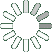Puzzle Command
Daily Puzzle
Number Logic #21
March 22, 2015Find a 6 digit number where:

The 1st digit is the number of 9s in the number.
The 2nd digit is an odd number.
The 3rd digit is a prime number.
The 4th digit is an even number.
The 5th digit is an odd number.
The 6th digit is any number.

Each digit is greater than the previous.

Puzzle Notes
6 digit numbers range from 100,000 to 999,999.
Enter in your solution below and click submit.